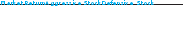### Create an Account

Home / Questions / Consider the following table which gives a security analyst’s expected return on two sto...

# Consider the following table which gives a security analyst’s expected return on two stocks for two particular market returns LO 7-2 5% 2% 3.5% 20 32 14 What are the betas of the two stocks

1. Consider the following table, which gives a security analyst’s expected return on two stocks for two particular market returns: (LO 7-2)

 5% 2% 3.5% 20 32 14

1. What are the betas of the two stocks?www.mhhe.com/bkm

2. What is the expected rate of return on each stock if the market return is equally likely to be 5% or 20%?

3. If the T-bill rate is 8%, and the market return is equally likely to be 5% or 20%, draw the SML for this economy.

4. Plot the two securities on the SML graph. What are the alphas of each?

5. What hurdle rate should be used by the management of the aggressive firm for a project with the risk characteristics of the defensive firm’s stock?If the simple CAPM is valid, which of the situations in Problems 13–19 below are possi- ble? Explain. Consider each situation independently.

Jun 17 2020 View more View LessSubscribe To Get Solution